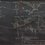# 2 original proofs that harmonic series diverges

1. All positive integers but $$1$$ are divisble by at least one prime. The probability an integer is divisble by $$p$$ is $$\frac{1}{p}$$; the complement of that is $$1-\frac{1}{p}$$ and so the product over all primes $$\displaystyle \prod_{p \ \text{prime}} \left( 1-\frac{1}{p} \right) = 0$$. From the Euler product, we thus know $$\zeta(1)$$ is divergent.

2. Assume the harmonic series converges; then it converges absolutely, since all its terms are positive. This is obviously a contradiction, since we can tell it is conditionally convergent from the ratio/root test.Note by Jake Lai
5 years, 1 month ago

This discussion board is a place to discuss our Daily Challenges and the math and science related to those challenges. Explanations are more than just a solution — they should explain the steps and thinking strategies that you used to obtain the solution. Comments should further the discussion of math and science.

When posting on Brilliant:

• Use the emojis to react to an explanation, whether you're congratulating a job well done , or just really confused .
• Ask specific questions about the challenge or the steps in somebody's explanation. Well-posed questions can add a lot to the discussion, but posting "I don't understand!" doesn't help anyone.
• Try to contribute something new to the discussion, whether it is an extension, generalization or other idea related to the challenge.

MarkdownAppears as
*italics* or _italics_ italics
**bold** or __bold__ bold
- bulleted- list
• bulleted
• list
1. numbered2. list
1. numbered
2. list
Note: you must add a full line of space before and after lists for them to show up correctly
paragraph 1paragraph 2

paragraph 1

paragraph 2

[example link](https://brilliant.org)example link
> This is a quote
This is a quote
    # I indented these lines
# 4 spaces, and now they show
# up as a code block.

print "hello world"
# I indented these lines
# 4 spaces, and now they show
# up as a code block.

print "hello world"
MathAppears as
Remember to wrap math in $$ ... $$ or $ ... $ to ensure proper formatting.
2 \times 3 $2 \times 3$
2^{34} $2^{34}$
a_{i-1} $a_{i-1}$
\frac{2}{3} $\frac{2}{3}$
\sqrt{2} $\sqrt{2}$
\sum_{i=1}^3 $\sum_{i=1}^3$
\sin \theta $\sin \theta$
\boxed{123} $\boxed{123}$

Sort by:

My proof for divergence requires no calculus.

$\dfrac {1}{1} + \dfrac {1}{2} + \dfrac {1}{3} + \dfrac {1}{4} + \dfrac {1}{5} + \dfrac {1}{6} + \dfrac {1}{7} + \dfrac {1}{8} + \ldots > \dfrac {1}{1} + \dfrac {1}{2} + \dfrac {1}{4} + \dfrac {1}{4} + \dfrac {1}{8} + \dfrac {1}{8} + \dfrac {1}{8} + \dfrac {1}{8} + \ldots$

$\dfrac {1}{1} + \dfrac {1}{2} + \dfrac {1}{3} + \dfrac {1}{4} + \dfrac {1}{5} + \dfrac {1}{6} + \dfrac {1}{7} + \dfrac {1}{8} + \ldots > \dfrac {1}{1} + \dfrac {1}{2} + \left( \dfrac {1}{4} + \dfrac {1}{4} \right) + \left(\dfrac {1}{8} + \dfrac {1}{8} + \dfrac {1}{8} + \dfrac {1}{8} \right) + \ldots$

$\dfrac {1}{1} + \dfrac {1}{2} + \dfrac {1}{3} + \dfrac {1}{4} + \dfrac {1}{5} + \dfrac {1}{6} + \dfrac {1}{7} + \dfrac {1}{8} + \ldots > \dfrac {1}{1} + \dfrac {1}{2} + \dfrac {1}{2} + \dfrac {1}{2} + \ldots$

$\dfrac {1}{1} + \dfrac {1}{2} + \dfrac {1}{3} + \dfrac {1}{4} + \dfrac {1}{5} + \dfrac {1}{6} + \dfrac {1}{7} + \dfrac {1}{8} + \ldots > \infty$

Hence proven.

- 5 years, 1 month ago

Oresme reincarnate!

- 5 years, 1 month ago

I'm surprised I know his name. Wasn't Nicole Oresme an astronomer, a mathematician and physicist?

- 5 years, 1 month ago

Your proof is often attributed to Oresme.

- 5 years, 1 month ago

Oh

- 5 years, 1 month ago

Right! This is the classical proof of divergence of harmonic series using comparison. This proof is also featured in the Wikipedia page on Harmonic series. Hence, not an original proof.

- 5 years, 1 month ago

Ye, but nonetheless, easy to remember.

- 5 years, 1 month ago

Your proof #1 just went over my head since I'm not quite familiar with Euler product and stuff yet. So, I won't comment on that.

Regarding your proof #2, how can you "tell it is conditionally convergent from the ratio/root test" ? Both ratio and root tests can only be used to test either absolute convergence or divergence. In other cases when the criteria for divergence or absolute convergence is not met, the test is inconclusive. When the tests are inconclusive, the sum can be convergent (absolute / conditional) or divergent. You cannot say for sure that the series must be conditionally convergent using ratio / root test in such inconclusive cases (as far as I know). I'm saying all this based on the Wikipedia articles on ratio / root tests.

Correct me if I'm wrong.

- 5 years, 1 month ago